# Circular Reference in ExcelArticle byJeevan A Y## What Is Circular Reference In Excel?

A circular reference is when a formula creates a direct or indirect reference to its cell. As a result, it may show the calculations erroneously. Due to this infinite cycle, the worksheet may begin to slow down.

For instance, we have some values in cells A2:A8 like the following image:

If we apply the SUM function in cell A9, we will get a circular reference error message.

=SUM(A2:A9)

Since we enter the SUM excel function in cell A9, the SUM function cannot include the same cell reference. So, it created a circular reference, and the result will be null.

###### Key Takeaways
• A circular reference occurs when a formula produces a direct or indirect reference to its cell.
• It requires the iterative calculation to be enabled to work; otherwise, it will return zero.
• Using the status bar and error checking options, we can find circular reference cells in the worksheet. Therefore, the iterative calculation should be disabled to make a status bar to show circular reference cell addresses.
• Circular references slow down the performance of the workbook.

### How To Use Circular Reference In Excel?

Assume we are calculating the cost associated with manufacturing a particular product. We have applied the SUM function in the B7 cell.

However, this is not the total cost because we still need to calculate profit in cell B6, and this will be 25% of the total cost arrived in cell B7.

Let us apply the formula in cell B6 as shown in the following image.

We have entered the formula =B7*25% and ended up getting a circular reference, as we can see in the preceding image.

However, this can be fixed with some changes in Excel settings, and we will see the below result:

The total cost is 217, 25% of the total cost.

Note: We will discuss the settings change later in this article.

### Enable/Disable Iterative Calculations in Excel?

By default, iterative calculations are disabled in Excel. To make the circular references work in the Excel workbook, you need to enable iterative calculations.

Follow the below steps to enable iterative calculations.

Step 1: Click on the “File” tab.

Step 2: Now click on “Options.”

Step 3: Go to “Formulas.”

Step 4: Under Calculation Options, check the Enable iterative calculation box.

Step 5: When you turn on iterative calculation, you need to set two more options:

• Maximum Iterations: You need to specify the number of times you need to perform recalculation in this box.
• Maximum Change: You need to specify the changes between each time recalculation happens in this box. The smaller the number, the more accurate result you get and the more time Excel takes to calculate the worksheet.

Maximum Iteration is set to 100, while Maximum Change is set to 0.001. It indicates Excel will cease calculating the circular formula after 100 iterations or if the difference between iterations is less than 0.001, whichever occurs first.

### How To Find Circular References In Excel?

We can find circular reference in Excel in two ways:

#### Option 1: Using Formula Tab

Follow the steps to find circular reference:

1. Go to the Formula tab.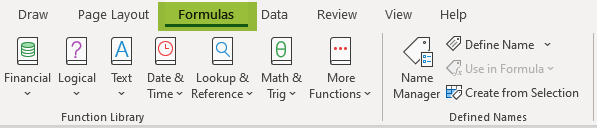2. Under Formula Auditing, click on the Error Checking drop-down.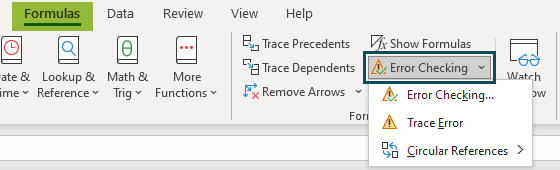3. Once you click on the drop-down, you have an option called Circular References. Hover on this, displaying all the recent circular references entered.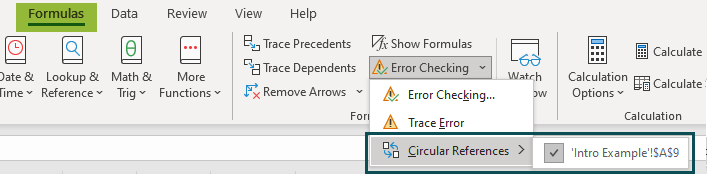The last circular reference entered is in cell A9.

#### Option 2: Using Status Bar

The status bar will display the circular reference cells if the worksheet has circular references.

Note: The status bar will only show circular reference in excel cells if the iteration calculation is disabled.

### Why Should Circular References Be Avoided?

Circular references are strongly recommended not to be used for the following reasons:

• A warning message will be displayed every time opening the new workbook if iterative action is not enabled.
• Circular references will lead to performance issues in the workbook and considerably slow down.
• Often circular references will lead to undesired or inaccurate results.

Therefore, design your Excel worksheet so that the necessity of circular references should not arise.

### Removing Circular References

Unfortunately, Excel has no straightforward way to remove circular reference in excel with just a click.

To eliminate the circular references, we need to inspect every circular reference cell using the steps shown above and remove it manually. We can also replace it with alternative formulas.

However, we can trace relationships between formula cells using the Formula Auditing option in Excel.

Formulas  > Formula Auditing

###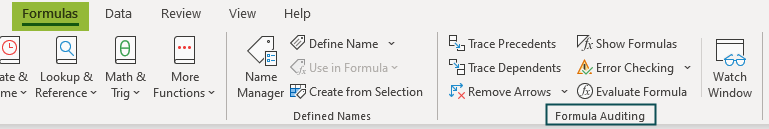Under Formula Auditing, we have two options Trace Precedents and Trace Dependents.

Trace Precedents: This will trace all the cells included in the formula cell and draw an arrow for them.

For example, look at the following image:

Cell B7 has SUM function, which takes references from cells B2:B6. To trace this, select cell B7 and click on the Trace Precedents option to draw an arrow-like shown in the following image.

As shown in the above image, an arrow from B2:B6 points toward cell B7, which means cell B7 takes the reference of cell B2:B6.

To perform this exercise, we can also use the following shortcut key.

Or,

Trace Dependents: Similarly, we can also trace cells dependent on specific cells. For instance, look at the following image.

By selecting cell B7, we clicked on Trace Dependents options. It shows an arrow towards cell B6, which means the value in cell B6 depends on the value arriving in cell B7.

To perform this exercise, we can also use the following shortcut key.

Or,

Like this, we can trace the relationship of cells in Excel formulas.

### Important Things To Note

• Circular references are recommended not to use, as it creates many problems if something goes wrong.
• We need to enable the iterative calculation option to make the circular reference work.
• Excel keeps giving the user a warning message whenever they open the workbook if the iterative calculation option is not enabled.

### Frequently Asked Questions (FAQs)

How to fix circular reference in excel?

Though it is hard to check circular reference in excel, we can still fix this by using some tricks.

For instance, look at the following image.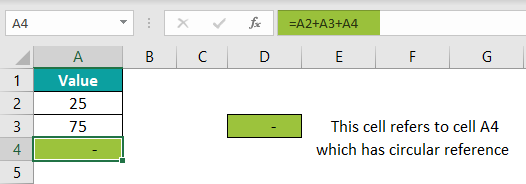Cell A4 has the formula A2+A3+A4. So, cell A4 refers to its cell, thus creating a circular reference.

And in cell D3, we have a formula that references cell A4.

Because cell A4 returns a blank cell, D4 also returns a blank.

To fix this, find the Circular Reference cell under Error Checking.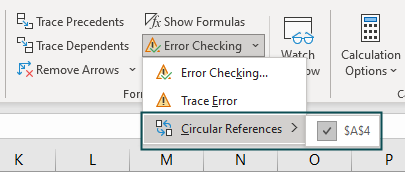As it shows, cell A4 is the circular reference cell. Therefore, edit the formula and remove circular reference in excel in cell A4.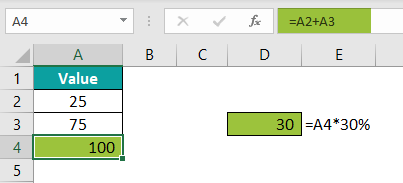We also have a fixed circular reference formula in cells A4 and D3; that value comes correctly.

How to see circular reference in excel?

Circular references can be seen in two ways:

Go to the Formula tab, click on the Error Checking drop-down list, and hover on Circular References; it will display all the circular referenced cells.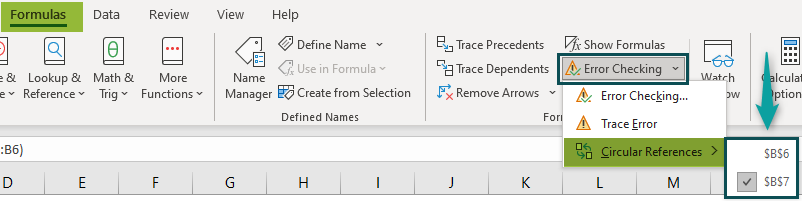Another way we can find it is by using the status bar. In the status bar, it will display circular referenced cells.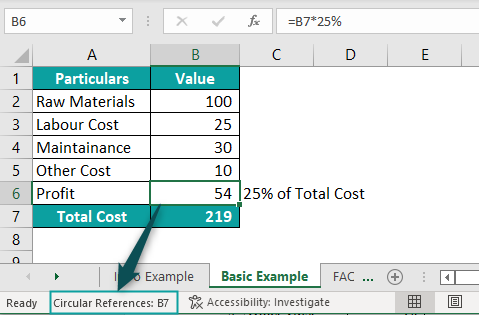How to allow circular reference in excel?

Circular references will work if the iterative calculation is enabled in Excel. Follow the below steps to enable iterative calculations in Excel.

File >>> Options >>> Formulas

Under Calculation Options, check the box of Enable iterative calculation.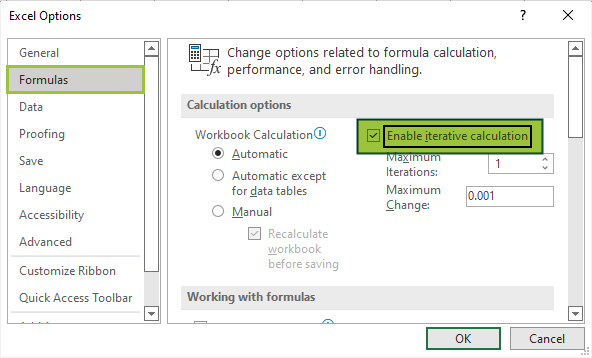How to use Circular Reference in Excel?

Circular references are strongly recommended not to use. However, we can use this option in Excel by activating the iterative calculation option.